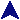IteratorsRuby Example Code# Here's a different way to add up an array.
fred = [ 4, 19, 3, 7, 32 ]
sum = 0
fred.each { |i| sum += i }
print "Sum of [", fred.join(" "), "] is #{sum}\n"

# Or create a secret message.
key = { 'A' => 'U', 'B' => 'Q', 'C' => 'A', 'D' => 'F', 'E' => 'D', 'F' => 'K',
'G' => 'P', 'H' => 'W', 'I' => 'N', 'J' => 'L', 'K' => 'J', 'L' => 'M',
'M' => 'S', 'N' => 'V', 'O' => 'Y', 'P' => 'O', 'Q' => 'Z', 'R' => 'T',
'S' => 'E', 'T' => 'I', 'U' => 'X', 'V' => 'B', 'W' => 'G', 'X' => 'H',
'Y' => 'R', 'Z' => 'C' }
print "\nThe encoded message is: "
"The secret message".each_byte do | b |
b = b.chr.upcase
if key.has_key?(b) then
print key[b]
else
print b
end
end
print "\n"

# But give us the info to read it anyway.
print "The key is: "
ct = 8
key.each { | k, v |
if ct == 8 then
print "\n   "
ct = 0
else
print ", "
end
ct = ct + 1
print "#{v} => #{k}"
}
print "\n\n"

# Some interesting things from Integer.
3.times { print "Hi! " }
print "\n"

print "Count: "
3.upto(7) { |n| print n, " " }
print "\n"
See:Ruby User's GuideRuby Manual 

Ruby iterators are methods which take and run a block of code. The block can be delimited by curly braces or by the keywords do and end. The brackets have higher precedence, and variables declared in them are destroyed when the bracked code exits.

The parameter names for the block are listed between | symbols at the start of the block. This syntax is borrowed from Smalltalk.

Note: Ruby uses the term "iterator" rather differently than C++.